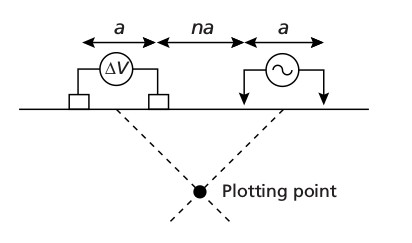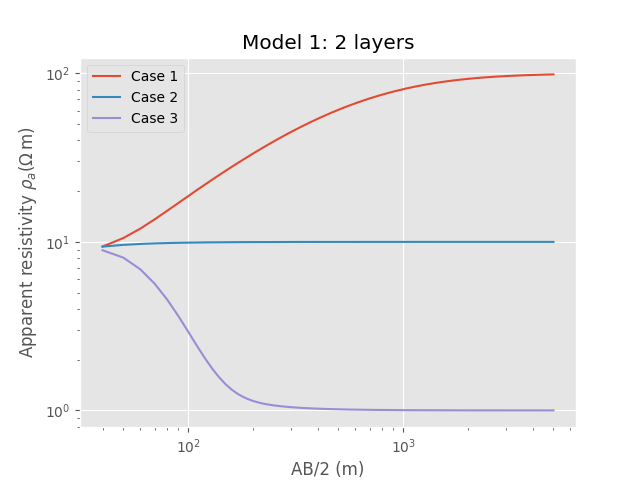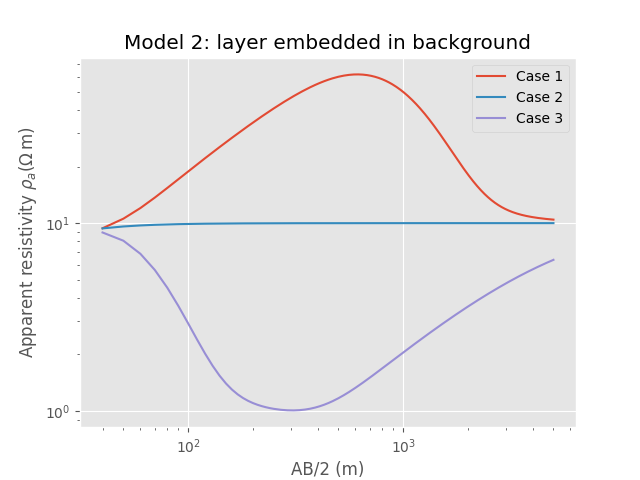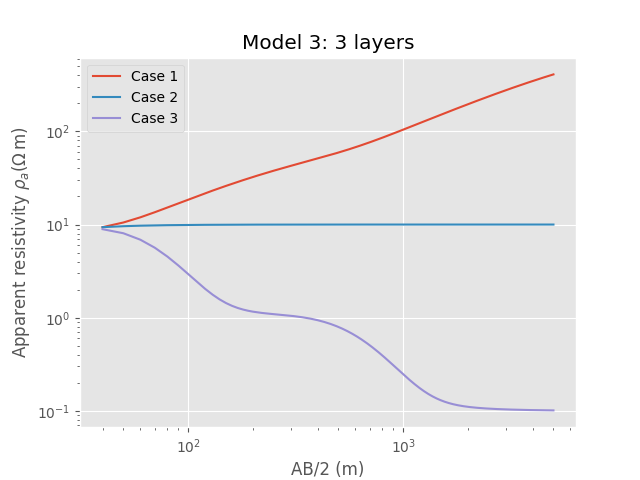# DC apparent resistivity#

DC apparent resistivity, dipole-dipole configuration.

There are various DC sounding layouts, the most common ones being Schlumberger, Wenner, pole-pole, pole-dipole, and dipole-dipole, at which we have a look here.

Dipole-dipole layout as shown in figure 8.32 in Kearey et al. (2002):The apparent resistivity $$\rho_a$$ of the plotting point is then computed with

$\rho_a = \frac{V}{I} \pi na(n+1)(n+2)\ ,$

where $$V$$ is measured Voltage, $$I$$ is source strength, $$a$$ is dipole length, and $$n$$ is the factor of source-receiver separation.

References

Kearey, P., M. Brooks, and I. Hill, 2002, An introduction to geophysical exploration, 3rd ed.: Blackwell Scientific Publications, ISBN: 0 632 04929 4.

import empymod
import numpy as np
import matplotlib.pyplot as plt
plt.style.use('ggplot')


## Compute $$\boldsymbol{\rho_a}$$#

First we define a function to compute apparent resistivity for a given model and given source and receivers.

def comp_appres(depth, res, a, n, srcpts=1, recpts=1, verb=1):
"""Return apparent resistivity for dipole-dipole DC measurement

rho_a = V/I pi a n (n+1) (n+2).

Returns die apparent resistivity due to:
- Electric source, inline (y = 0 m).
- Source of 1 A strength.
- Source and receiver are located at the air-interface.
- Source is centered at x = 0 m.

Note: DC response can be obtained by either t->infinity s or f->0 Hz. f = 0
Hz is much faster, as there is no Fourier transform involved and only
a single frequency has to be computed. By default, the minimum
frequency in empymod is 1e-20 Hz. The difference between the signals
for 1e-20 Hz and 0 Hz is very small.

For more explanation regarding input parameters see empymod.model.

Parameters
----------
depth : Absolute depths of layer interfaces, without air-interface.
res : Resistivities of the layers, one more than depths (lower HS).
a : Dipole length.
n : Separation factors.
srcpts, recpts : If < 3, bipoles are approximated as dipoles.
verb : Verbosity.

Returns
-------
rho_a : Apparent resistivity.
AB2 : Src/rec-midpoints

"""

# Get offsets between src-midpoint and rec-midpoint, AB
AB = (n+1)*a

# Collect model, putting source and receiver slightly (1e-3 m) into the
# ground.
model = {
'src': [-a/2, a/2, 0, 0, 1e-3, 1e-3],
'rec': [AB-a/2, AB+a/2, AB*0, AB*0, 1e-3, 1e-3],
'depth': np.r_[0, np.array(depth, ndmin=1)],
'freqtime': 1e-20,  # Smaller f would be set to 1e-20 be empymod.
'verb': verb,       # Setting it to 1e-20 avoids warning-message.
'res': np.r_[2e14, np.array(res, ndmin=1)],
'strength': 1,      # So it is NOT normalized to 1 m src/rec.
'htarg': {'pts_per_dec': -1},
}

return np.real(empymod.bipole(**model))*np.pi*a*n*(n+1)*(n+2), AB/2


## Plot-function#

Second we create a plot-function, which includes the call to comp_appres, to use for a couple of different models.

def plotit(depth, a, n, res1, res2, res3, title):
"""Call comp_appres and plot result."""

# Compute the three different models
rho1, AB2 = comp_appres(depth, res1, a, n)
rho2, _ = comp_appres(depth, res2, a, n)
rho3, _ = comp_appres(depth, res3, a, n)

# Create figure
plt.figure()

# Plot curves
plt.loglog(AB2, rho1, label='Case 1')
plt.plot(AB2, rho2, label='Case 2')
plt.plot(AB2, rho3, label='Case 3')

# Legend, labels
plt.legend(loc='best')
plt.title(title)
plt.xlabel('AB/2 (m)')
plt.ylabel(r'Apparent resistivity $\rho_a (\Omega\,$m)')

plt.show()


### Model 1: 2 layers#

layer

depth (m)

resistivity (Ohm m)

air

$$-\infty$$ - 0

2e14

layer 1

0 - 50

10

layer 2

50 - $$\infty$$

100 / 10 / 1

plotit(
50,                 # Depth
20,                 # a (src- and rec-lengths)
np.arange(3, 500),  # n
[10, 100],          # Case 1
[10,  10],          # Case 2
[10,   1],          # Case 3
'Model 1: 2 layers')### Model 2: layer embedded in background#

layer

depth (m)

resistivity (Ohm m)

air

$$-\infty$$ - 0

2e14

layer 1

0 - 50

10

layer 2

50 - 500

100 / 10 / 1

layer 3

500 - $$\infty$$

10

plotit(
[50, 500],          # Depth
20,                 # a (src- and rec-lengths)
np.arange(3, 500),  # n
[10, 100, 10],      # Case 1
[10,  10, 10],      # Case 2
[10,   1, 10],      # Case 3
'Model 2: layer embedded in background')### Model 3: 3 layers#

layer

depth (m)

resistivity (Ohm m)

air

$$-\infty$$ - 0

2e14

layer 1

0 - 50

10

layer 2

50 - 500

100 / 10 / 1

layer 3

500 - $$\infty$$ | 1000 / 10 / 0.1

plotit(
[50, 500],          # Depth
20,                 # a (src- and rec-lengths)
np.arange(3, 500),  # n
[10, 100, 1000],    # Case 1
[10,  10,   10],    # Case 2
[10,   1,    0.1],  # Case 3
'Model 3: 3 layers')empymod.Report()

 Wed Aug 31 16:00:36 2022 UTC OS Linux CPU(s) 2 Machine x86_64 Architecture 64bit RAM 7.6 GiB Environment Python File system ext4 Python 3.8.6 (default, Oct 19 2020, 15:10:29) [GCC 7.5.0] numpy 1.22.4 scipy 1.9.1 numba 0.56.0 empymod 2.2.0 IPython 8.4.0 matplotlib 3.5.3

Total running time of the script: ( 0 minutes 2.015 seconds)

Estimated memory usage: 9 MB

Gallery generated by Sphinx-Gallery